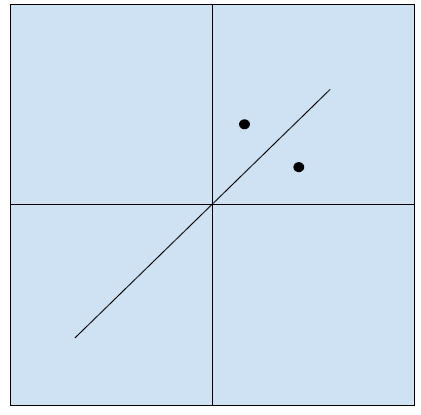# Find mirror image of a point in 2-D plane in C++

In this problem, we are given a point P in a 2-D plane and the points a, b, c of the equation ax + by + c = 0. Our task is to find a mirror image of a point in 2-D plane.

Let’s take an example to understand the problem,

## Input

P = (2, 1), a = 1, b = -1, c = 0

## Output

(1, 2)

## Explanation

The plane looks like,## Solution Approach

To solve the problem, we need to find the equation point P' with coordinates (x', y'). So, we have R, the midpoint where the line form P - P' intersects the mirror line.

The line P-R-P' is perpendicular to the mirror. Hence, the equation of line will be,

ay - by + d = 0

The points are P(x, y) ; P'(x', y') ; R(xm, ym).

The points P and R are known. So, using the equations we will find P’ as,

$$\left(\frac{??'-??}{??}\right)=\left(\frac{??'-??}{??}\right)=\left(\frac{????-????+??}{??^2+x^2}\right)$$

Program to illustrate the working of our solution,

## Example

Live Demo

#include <iostream>
using namespace std;
void findMirrorImage( double a, double b, double c, double x, double y){
double points = -2 * (a * x + b * y + c) / (a * a + b * b);
double xm = points * a + x;
double ym = points * b + y;
cout<<"("<<xm<<","<<ym<<")";
}
int main(){
double a = -1.0;
double b = 1.0;
double c = 0.0;
double x = 1.0;
double y = 0.0;
cout<<"Image of point ("<<x<<", "<<y<<") using mirror ("<<a<<")x + ("<<b<<")y + ("<<c<< ") = 0, is :";
findMirrorImage(a, b, c, x, y);
return 0;
}

## Output

Image of point (1, 0) using mirror (-1)x + (1)y + (0) = 0, is :(0,1)